## 2.4.2 Converting to the State-Space Representation

It is useful to characterize the relationship between Formulation 2.4 and the original formulation of discrete feasible planning, Formulation 2.1. One benefit is that it immediately shows how to adapt the search methods of Section 2.2 to work for logic-based representations. It is also helpful to understand the relationships between the algorithmic complexities of the two representations.

Up to now, the notion of state'' has been only vaguely mentioned in the context of the STRIPS-like representation. Now consider making this more concrete. Suppose that every predicate hasarguments, and any instance could appear in each argument. This means that there are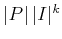complementary pairs, which corresponds to all of the ways to substitute instances into all arguments of all predicates. To express the state, a positive or negative literal must be selected from every complementary pair. For convenience, this selection can be encoded as a binary string by imposing a linear ordering on the instances and predicates. Using Example 2.6, the state might be specified in order as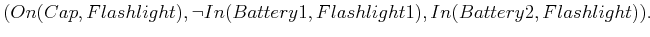(2.25)

Using a binary string, each element can be 0'' to denote a negative literal or 1'' to denote positive literal. The encoded state is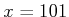for (2.25). If any instance can appear in the argument of any predicate, then the length of the string is. The total number of possible states of the world that could possibly be distinguished corresponds to the set of all possible bit strings. This set has size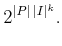(2.26)

The implication is that with a very small number of instances and predicates, an enormous state space can be generated. Even though the search algorithms of Section 2.2 may appear efficient with respect to the size of the search graph (or the number of states), the algorithms appear horribly inefficient with respect to the sizes ofand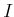. This has motivated substantial efforts on the development of techniques to help guide the search by exploiting the structure of specific representations. This is the subject of Section 2.5.

The next step in converting to a state-space representation is to encode the initial state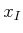as a string. The goal set,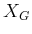, is the set of all strings that are consistent with the positive and negative goal literals. This can be compressed by extending the string alphabet to include a don't care'' symbol,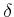. A single string that has a 0'' for each negative literal, a 1'' for each positive literal, and a'' for all others would suffice in representing anythat is expressed with positive and negative literals.

Now convert the operators. For each state,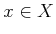, the set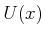represents the set of operators with preconditions that are satisfied by. To apply the search techniques of Section 2.2, note that it is not necessary to determineexplicitly in advance for all. Instead,can be computed whenever eachis encountered for the first time in the search. The effects of the operator are encoded by the state transition equation. From a given, the next state,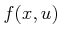, is obtained by flipping the bits as prescribed by the effects part of the operator.

All of the components of Formulation 2.1 have been derived from the components of Formulation 2.4. Adapting the search techniques of Section 2.2 is straightforward. It is also straightforward to extend Formulation 2.4 to represent optimal planning. A cost can be associated with each operator and set of literals that capture the current state. This would express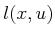of the cost functional,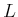, from Section 2.3. Thus, it is even possible to adapt the value-iteration method to work under the logic-based representation, yielding optimal plans.

Steven M LaValle 2020-08-14